# Cross And Dot Products

The age old answer, what the heck does :Cross() and :Dot() do? Not many tutorials online specified towards roblox show any results so I thought I might as well cover it. Before we start though I’d like to say this is an import from my website. All I have done is made it for the devforum. Anyways let’s get started on -

## Cross and Dot Products

A dot product refers to the scalar product of two vectors. In a sense it means the combined length. This length can also be expressed as the length of the adjacent leg when viewing the unit circle and assuming both of our vectors are normalized. It is useful for checking the angle between two vectors. Additionally, a cross product isn’t a scalar product but rather a new vector. Cross products will fall into the third dimension if you do this in cartesian space but in a sense what you are doing is getting the vector which is perpendicular to your vectors and setting its length to be the amount of area that the two vectors take up. Whether this new cross product vector should point in the negative or positive is dependent on how you multiplied them.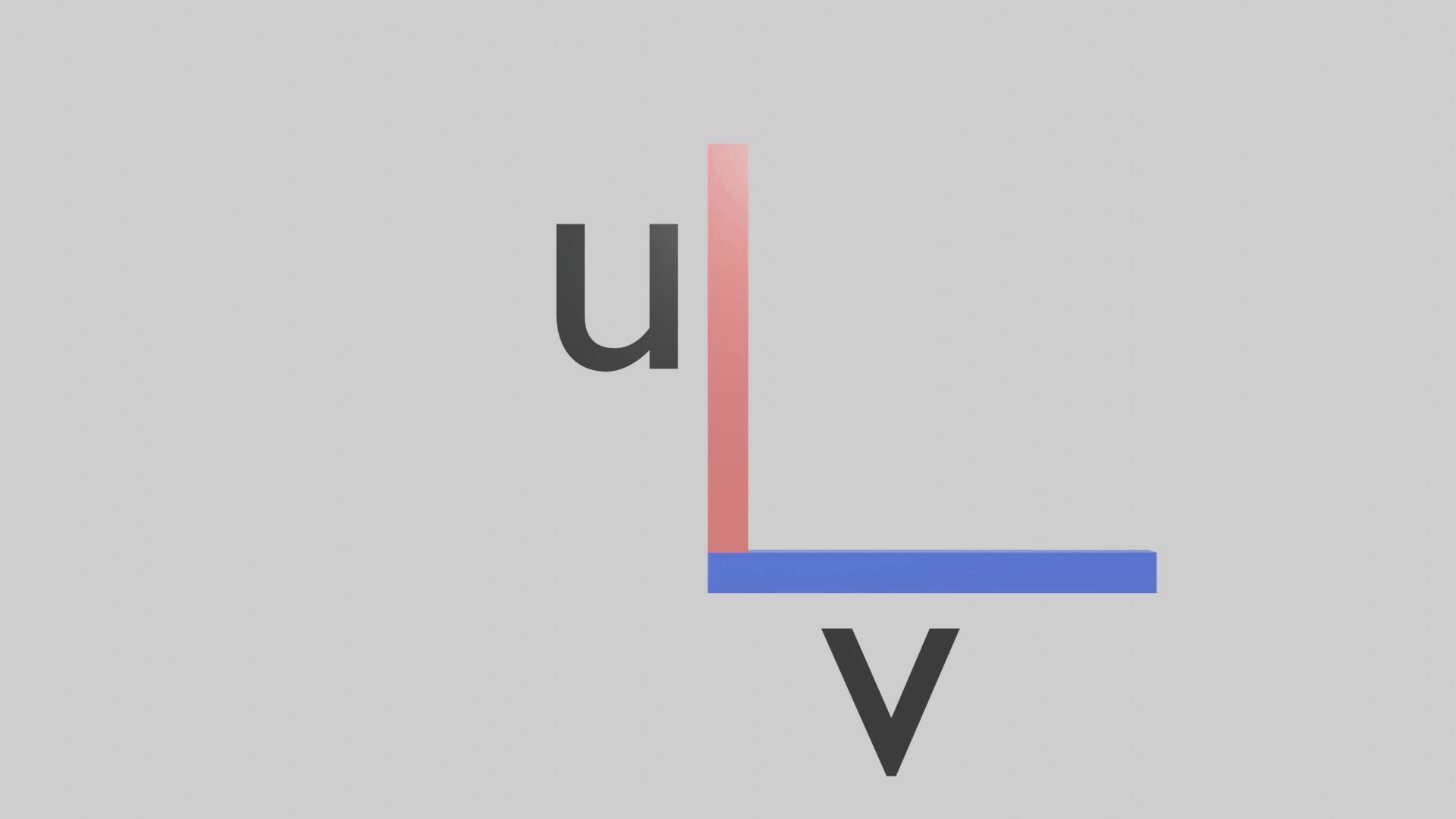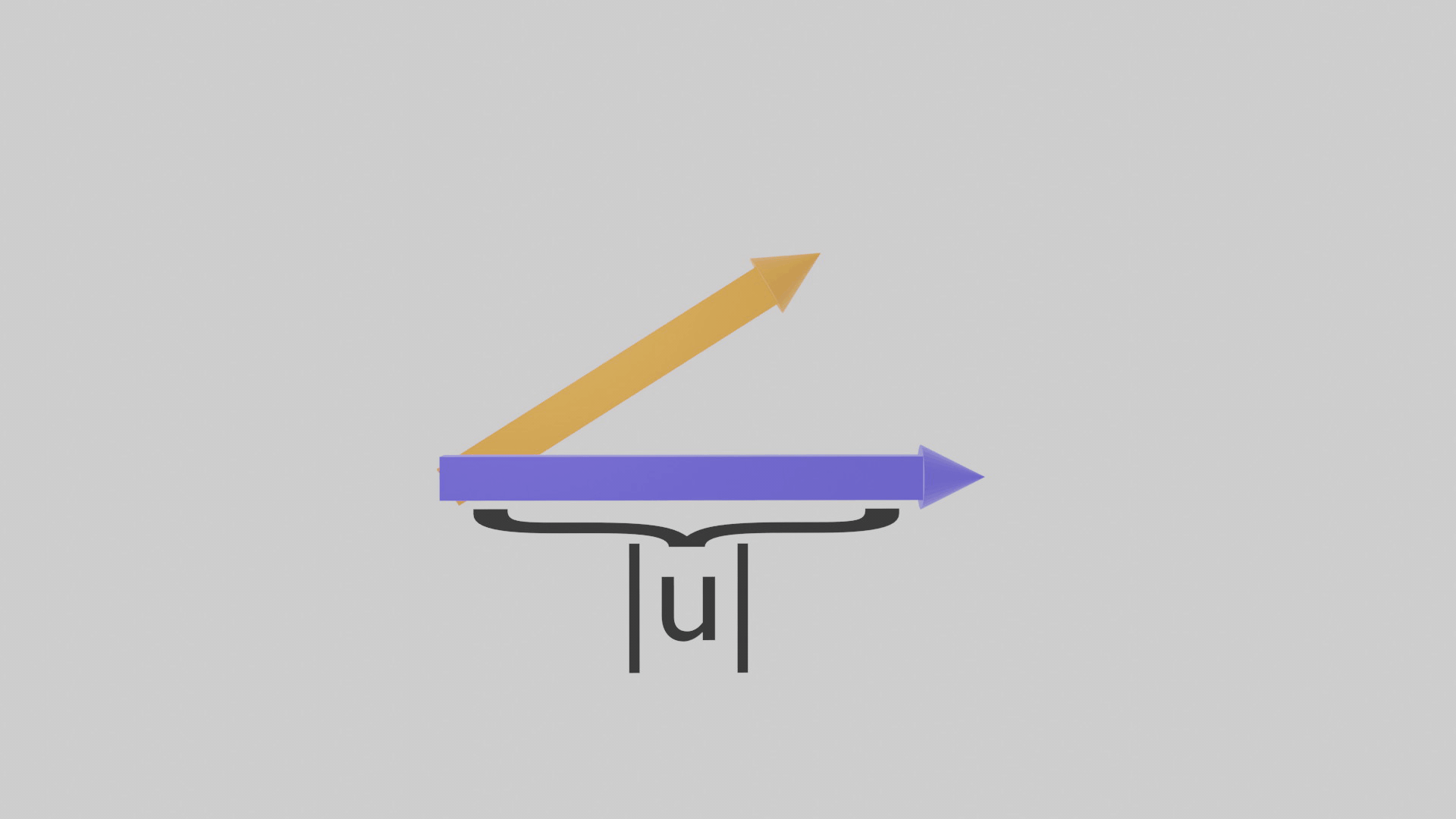A common problem seen in game developers when implementing mechanics which involve hit detection, whether that is a axe cutting system or a punching system is that the object which is giving the damage in a sense needs to be extremely precise in order for it to register a hit. This can lead to players having to go in first person for example in order to play your game. A solution for this while still retaining the mechanic is with dot products.

(it was too big to upload here but it was basically a gif of a tree cutting mechanic from my game)

Suppose we want to detect if an object is facing another object within a field of view while also being a given distance close to it. Let’s rename these objects to be female for the object detecting damage and male for the object giving damage. We will also define two vectors. One being the males front facing vector; or in other words the LookVector and the other being derived from the normalized vector where the tail is the males position and the head being the females position. To return a uniform value of this dot product we first need to normalize the vectors. I am going to explain the math behind calculating dot products from scratch however do note, your game engine or programming language has a very high chance of already having these functions built in. So do your homework first! Anyways for all of you OpenCL students let’s dive in.If you watched my last video on matrices I mentioned how to get the dot product of 2 matrices. The process is the same for vectors however you don’t transpose the data. Again, we want to normalize or in a sense make our vectors length 1 while also maintaining direction. The way we do this is first we need to calculate its length. We can derive this by abstracting from the pythagorean theorem. Well actually it’s the exact same except we are adding another variable, that being z. Where a is now x and b is now y. Putting it all together to calculate the length of a vector where x,y,z represent its components that is;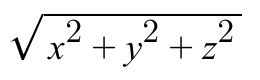Now that we know our vector’s magnitude we can use this and divide it by every component in our vector to find what our components should be.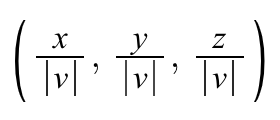With our new unit vectors we multiply each one of its components correspondent to the others position. Here is an example. The normalized vectors in this case were (1/√5, 2/√5) and (1/√2, 1/√2). Processing this we get a number which is roughly 0.95.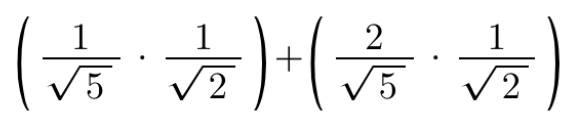We can also describe the dot product as the product of their magnitudes times the cosine of the angle between them. However we do it this way instead as the angle measure is usually unknown. However knowing that dot products can be described as this we can infer that the angle measure is directly linked with the unit circle. This makes our lives a lot easier as now if we want to know the ratio for any angle between two vectors we can simply put our calculator in degrees mode and do cosine of the given angle measure. So if you want a little chart of the ratios of the dot products for unit vectors just look at the x value of the unit circle. Note we are in a range within 0 to 180 degrees.

Why is the dot product linked with the unit circle? Well dot products 3d or not can be applied in a sense where they respect cartesian space. Not only that but since we know that the cosine of any given function will be its adjacent over its hypotenuse we can also infer that our adjacent in this case is the x axis with our hypotenuse being the vector formed from our male and female vector.

Cross products are interesting. As we know they take in 2 vectors and multiply them in a special way to get the cross product. I think it also should be noted when we say cross product or dot product we are referring to the actual answer we get when applying the cross and dot algorithms in a sense to our vectors. We first need to know what way our cross product should be facing. The right hand rule I think is the easiest way to visualize this. Stick out your hand so that you form a gun with your index finger and thumb. We will name your index finger “u”. Now stick out your middle finger so that it is perpendicular to your thumb and u. Your middle finger is “v”. Multiplying u first, then v will tell us our thumb is the cross products direction.

Note though if you multiplied v first the direction would be the other way, not up but rather down. Now that we have our cross products direction we normalize it so that we can apply scalar multiplication, where the magnitude is equivalent to the area of both of the other vectors. From this we can infer that the cross products magnitude is the biggest when the two vectors are 90 degrees respective to each other. The reason why is because the two multiples form a square, ensuring the most overall area. Like I said with the dot product cross products usually have their inbuilt function in the game engine you use but here are some ways you can calculate them. We learned that the dot product can give us the angle of two vectors. More specifically if we get its arc cosine of the new given ratio we know we can find it.

So to find the angle of two given cross products now what we do is we first get the dot product, note we should skip the normalization step this time. From there we get its inverse cosine, or arc cosine in other words. This will tell us the degree difference of the two given vectors. From there we get the product of both of their magnitudes as if we were doing a standard find the area of a rectangle problem. Then we multiply that by the sine of our angle. Remember sine takes in radians so if you can go into degrees mode or multiply it by pi/180. From there we set the components of our normalized vector cross product to be perpendicular to them. To understand why we use sine it’s because we are solving an angle measure which we don’t know. That being how much of our square derived from getting the product of them should be extended while also maintaining its area. This is now our scalar, from there it is simply multiplying the vector by the unit vector.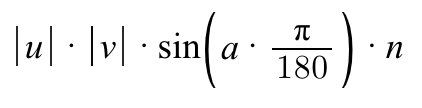Dot products are useful for determining if an enemy is facing a player for example. Cross products are not used as much in game development. However they do have a lot of uses in equations such as calculating the torque force. Understanding how these methods of multiplication work on a deep level and not just memorizing some equations helps us with implementing them in our workflow. Here is some homework for y’all. Using what you now know, create a video game where if you face an object within a certain field of view, it will turn your screen red.

If you guys are still confused the video version is here

24 Likes

Thanks! My gun will be way better using these tricksGreat tutorial, but an easier way to explain dot product to beginners is as a scalar projection of one vector onto another vector (which is also why it is sometimed called a projection product).

1 Like

I know in reality the actual dot product is the ratio of them. I realize this now and im going to make a better visualization.

Note cos() isnt needed

There I updated the correct version, my bad